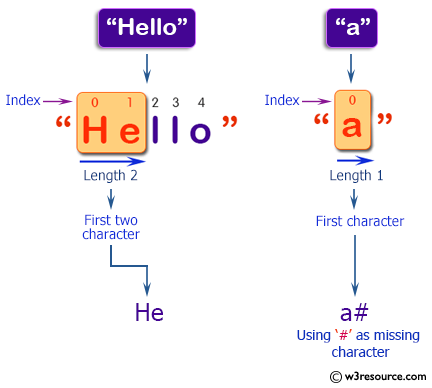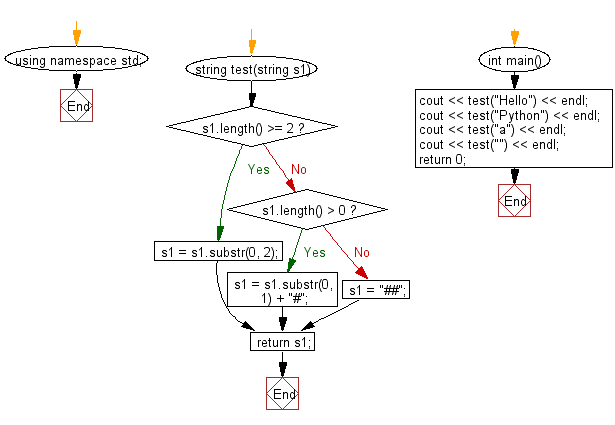﻿ C++ : New string, using first two characters of a string# C++ Exercises: Create a new string of length 2, using first two characters of a given string

## C++ Basic Algorithm: Exercise-71 with Solution

Write a C++ program to create a new string of length 2, using first two characters of a given string. If the given string length is less than 2 use '#' as missing characters.

Sample Solution:

C++ Code :

``````#include <iostream>

using namespace std;

string test(string s1)
{
if (s1.length() >= 2)
{
s1 = s1.substr(0, 2);
}
else if (s1.length() > 0)
{
s1 = s1.substr(0, 1) + "#";
}
else
{
s1 = "##";
}

return s1;
}
int main()
{
cout << test("Hello") << endl;
cout << test("Python") << endl;
cout << test("a") << endl;
cout << test("") << endl;
return 0;
}
``````

Sample Output:

```He
Py
a#
##
```

Pictorial Presentation:Flowchart:C++ Code Editor:

Contribute your code and comments through Disqus.

What is the difficulty level of this exercise?

﻿